• 任意从键盘输入一组数字，然后将这些数字按顺序排列
• 拿出列表里的第0个元素，它赋给一个叫做“最小值”的变量，然后用这个变量去跟后面的每个数字一一对比，如果碰到比它小的，就那个新发现的小数字赋给“最小值”变量，否则维持原状。 这样一轮下来，就能找到...
一、自己造轮子
第一种方式的思路：
拿出列表里的第0个元素，把它赋给一个叫做“最小值”的变量，然后用这个变量去跟后面的每个数字一一对比，如果碰到比它小的，就把那个新发现的小数字赋给“最小值”变量，否则维持原状。
这样一轮下来，就能找到当前这些数字里的最小值，也就是应该在升序的列表中排在队首的元素。而这一轮当中，我们进行了“”元素总个数-1”次比较。紧接着，把这个最小的元素从原列表中删除。再用同样的方法，找出剩下的数字里最小的。
列表里一共有10个数字的话，上述过程就应该重复9次。因为只剩下一个元素的时候就不需要比较了，它自然是最大的，排在队尾。
这种排序方法叫做“选择排序”。算法的复杂度是O（n²）。
lst=[2,56,4,9,17,30,7,8,24,1]
order=[]

for j in range(10,1,-1):
#j代表列表里当前的元素个数
smallest=lst
for i in range(1,j):
#i代表元素的索引，也就是拿第0个元素和列表里的第1个比较，第2个比较，一直比到第j-1个，完成一轮
if smallest<=lst[i]:
pass
else:
smallest=lst[i]

lst.remove(smallest)
#从原列表中移除已经确认的当前最小元素
order.append(smallest)
#把这个当前最小元素追加到升序列表中
order.append(lst)
#列表里最后剩下的那个元素追加到队尾
print(order)  
第二种方式的思路：
我们从列表中找到一个数字作为基准，然后去查看其它数字，比它小的放到一个新列表里，并把这个新列表放到基准数字的左边，比它大的数字放到另外一个列表里，并把这个装满大数字的列表放到基准数字的右边。一种非常理想的情况是，小数字列表里的元素本身就已经按照升序排好了，大数字列表里的元素也本身就按照升序排好了，那我们只要把小数字列表、基准数字、大数字列表拼接到一起就完成了全部数字的排序。而这个理想情况也是需要我们自己去实现的。我们要不断重复找基准数字、把其他数字分为小数字阵营和大数字阵营的工作，直到什么时候结束呢？直到最后切分出来的小数字/大数字列表里只有一个元素或一个元素都没有就可以停止了。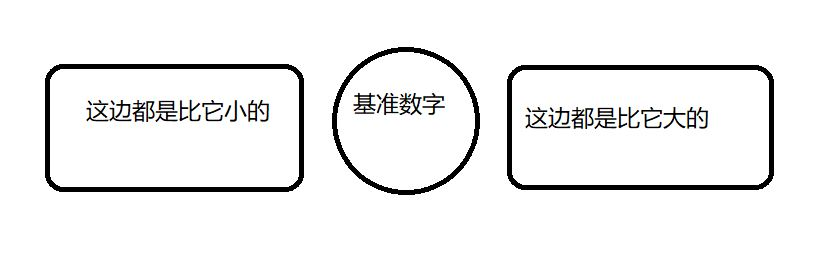现在我们有了基线条件，还有了需要不断重复的工作。一个词呼之欲出——递归！
其实这种排序方式就是“快速排序”。算法的复杂度是O（n log n）
def quicksort(arr):
'''快速排序函数'''
if len(arr)<2:
return arr
#上面是描述基线条件的部分，当列表里的元素不足两个时，直接原样返回
else:
pivot=arr
#把列表开头的元素当做基准数字
less=[]
greater=[]
#上面创建了用于存放小于基准数字和大于基准数字的元素的空列表
for i in arr[1:]:
if i<=pivot:
less.append(i)
else:
greater.append(i)
#上面是遍历列表里除掉基准数字以外的数字，按照我们的规定分别存放到两个列表里
return quicksort(less)+[pivot]+quicksort(greater)
#返回的就是我们需要的顺序，这里记得要把基准数字放到一个单独的列表里，否则拼接会失败

print(quicksort([23,9,55,33,24,15]))
#随便找个无序列表试一试我们的函数好不好用


二、用前人造好的轮子
lst=[2,56,4,9,17,30,7,8,24,1]
lst.sort()
#sort是列表的一个方法，默认会把列表里的数字按照升序排列
print(lst)
*********************************************************************************************************************
如果这篇博文帮到了你，就请给我点个赞吧(#^.^#)
有疑问也欢迎留言~博主可nice啦，在线秒回ヾ(◍°∇°◍)ﾉﾞ
展开全文python 升序 sort
• 其余列正常显示数据库中的数据，只有第一列显示按顺序排列数字，有多少行数据，就显示多少数字，类似于对数据的一个排名，但是这一列一定要有表头easyui
• matlab脚本，可以重命名一个文件夹下的多个同类型文件，重命名后按数字00001-000xx排列
• 遇到输出形式是set，但要求set里面的元素按顺序排列？ 概念 集合（set）是一个无序的不重复元素序列，可以使用大括号 { } 或者 set() 函数创建集合。 问题1 我尝试了一下在jupyter 里先将list排序再转换为set，如果...
遇到输出形式是set，但要求set里面的元素按顺序排列？
概念
集合（set）是一个无序的不重复元素序列，可以使用大括号 { } 或者 set() 函数创建集合。
问题1
我尝试了一下在jupyter 里先将list排序再转换为set，如果元素有异号数字的，只要是print结果，set的顺序都会改变。 但奇怪的是，如果只是利用python自己的特性输出，不用print，set的输出又是按顺序的。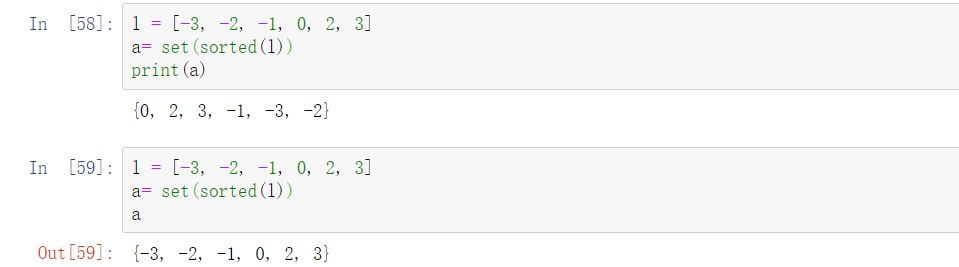解决方法
既然不能直接输出，那就改个方法print出来。
l = [-3, -4, -1, 0, 2, 3]
print('{', end = '')
print(', '.join(str(i) for i in l),end = '')
print('}')

输出：{-3, -4, -1, 0, 2, 3}
第一次写笔记，如果有更好的写法或者建议，请大家指教！
展开全文集合 python 排序
• 构建一个列表，分解的数字放进去。 创建函数，采用N%10，获得余数,将余数放进列表中。 采用N//10除去个位数（如1234//10 =123)，进行下一次递归。 result =[] def get_digits(n): if n > 0: ...
思想：
构建一个列表，把分解的数字放进去。  创建函数，采用N%10，获得余数,将余数放进列表中。  采用N//10除去个位数（如1234//10 =123)，进行下一次递归。 result =[] def get_digits(n): if n > 0: result.insert(0,n%10)#获得余数，并放进列表 get_digits(n//10)#除去个位数 get_digits(1034) print(result)
结果如下： [1, 0, 3, 4]
展开全文• 将字典顺序排列In this post, I want to show you a method to generate sorted permutations of a set. We will focus on generating these permutations in lexicographic or numeric order. There are ...

将字典按某顺序排列

In this post, I want to show you a method to generate sorted permutations of a set. We will focus on generating these permutations in lexicographic or numeric order. There are several ways to do this, but some are better than others in terms of efficiency.
在本文中，我想向您展示一种生成集合的排序排列的方法。 我们将重点介绍按字典顺序或数字顺序生成这些排列。 有几种方法可以做到这一点，但是就效率而言，有些方法比其他方法要好。
We’ll also look at a method to obtain a permutation at an arbitrary position in our lexicographically ordered sequence. With this method, we can generate the permutation we’re looking for more directly. We won’t need to generate any of the previous permutations in the sequence that come before our target one.
我们还将研究一种方法，以按字典顺序排列的任意位置获得置换。 使用这种方法，我们可以更直接地生成我们正在寻找的排列。 我们不需要按目标序列之前的顺序生成任何先前的排列。
Let’s start by refreshing our memory on what a permutation is.
让我们首先刷新一下排列是什么的记忆。
什么是排列？ (What Is a Permutation?)
From combinatorics, a permutation–also called “order”–is the number of rearrangements of elements in a set. The number of permutations on a set of n elements is given by n! (n factorial). For example, in the set {1, 2, 3} there are 3! = 3x2x1 = 6 permutations: {1 ,2, 3}, {1, 3, 2}, {2, 1, 3}, {2, 3, 1}, {3, 1, 2}, and {3, 2, 1}.
从组合学的角度来看，排列(也称为“顺序”)是一组元素重新排列的数量。 一组n个元素上的排列数由n！ (n阶乘)。 例如，在集合{1,2,3}中有3！ = 3x2x1 = 6个排列： {1、2、3}，{ 1、3、2 }，{ 2、1、3 }，{ 2、3、1 }，{ 3、1、2 }和{3， 2，1} 。
The number of ways to rearrange a subset of k elements out of a set of n elements is given by :
重新排列一组n个元素中的k个元素的子集的方式数目由下式给出：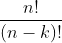This formula is easily derived from our previous definitions. Let’s follow a concrete example to illustrate this. Suppose we want to find the number of ways to arrange 4 books from a collection of 10. According to our formula above, we have:
该公式很容易从我们之前的定义中得出。 让我们来看一个具体的例子来说明这一点。 假设我们想从10本书的集合中找到整理4本书的方法的数目，根据上面的公式，我们有：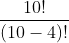We can think about this in the following way. In the numerator, we have the number of ways to arrange 10 books–that is 10!. But, we only care about the first 4 ways. So, we divide out the remaining 6 ways–or ( 10-4)! = 6!:
我们可以通过以下方式来考虑。 在分子中，我们有10种书的排列方法，即10种！ 。 但是，我们只关心前四种方式。 因此，我们将剩下的6种方式分开-或( 10-4)！ = 6！ ：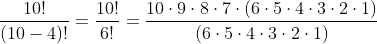Finally, keep in mind that in a permutation, we count all possible orderings in a set.
最后，请记住，在排列中，我们计算集合中所有可能的顺序。
产生排列 (Generating Permutations)
We can generate permutations using different methods. And, depending on the method we choose, the order of the resulting permutations will change. Also, some methods will be more efficient than others. Robert Sedgewick concluded in his survey paper Permutation Generation Methods that the fastest algorithm to generate permutations is Heap’s algorithm. However, the resulting rearrangements form this method are not in lexicographic order.
我们可以使用不同的方法生成排列。 并且，根据我们选择的方法，结果排列的顺序将改变。 而且，某些方法将比其他方法更有效。 罗伯特·塞奇威克(Robert Sedgewick)在其调查论文《 排列生成方法》中得出结论，生成排列最快的算法是Heap算法 。 但是，此方法产生的重排不是按字典顺序。
词典顺序排列 (Permutations in Lexicographic Order)
In our case, we want to list them in lexicographic-or numerical-order. As an example, let’s generate the permutations of the set {0 1 2}. We take the smallest number, 0, and put it at the front then we append the remaining 1 and 2. This gives us the first permutation {0 1 2}. Next, keeping 0 in front, we rearrange 1 and 2: {0 2 1}. We repeat the process, but now with 1 at the front. So, we obtain permutations {1 0 2} and {1 2 0}. Finally, we repeat with 2 at the front: {2 0 1} and {2 1 0}. The resulting permutations list is the following.
在我们的情况下，我们要按字典顺序或数字顺序列出它们。 例如，让我们生成集合{0 1 2}的排列。 我们取最小的数字0 ，并将其放在最前面，然后附加其余的1和2 。 这给了我们第一个排列{0 1 2}。 接下来，将0放在前面，我们重新排列1和2 ：{0 2 1}。 我们重复该过程，但是现在前面是1 。 因此，我们获得排列{1 0 2}和{1 2 0} 。 最后，我们在前面重复2 ： {2 0 1}和{2 1 0} 。 生成的排列列表如下。
1. {0 1 2} 2. {0 2 1} 3. {1 0 2} 4. {1 2 0} 5. {2 0 1} 6. {2 1 0}
Notice that the list is arranged in numerical order. In other words, each subsequent arrangement of the numbers is greater than the previous one.
请注意，列表按数字顺序排列。 换句话说，数字的每个后续排列都大于前一个。
In this example, we only had 6 permutations of the set {1 2 3}. So, it was easy to list them all. However, as the size of the set starts to grow, the number of permutations increases even faster. Remember that it follows a factorial rate of growth-the number of permutations of a set of size n = n!. So, if we wanted to generate the permutations of the set {0 1 2 3 4 5 6 7 8 9}, we will end up with 10! = 3,628,800 rearrangements of the set.
在此示例中，我们只有{1 2 3}集的6个置换。 因此，将它们全部列出很容易。 但是，随着集合的大小开始增长，排列的数量甚至更快地增加。 请记住，它遵循阶乘增长率-一组大小为n = n的排列数！ 。 因此，如果我们想生成集合{0 1 2 3 4 5 6 7 8 9}的置换，我们将得到10！ =该集合的3,628,800个重排。
A classic algorithm to generate permutations in lexicographic order is as follows. Consider the list of numbers s = [1, 2, 3, 4]. Notice that the sequence is initially sorted. This is required by the algorithm. Now–assuming the indexing of the list is zero-based-we proceed as follows:
生成按字典顺序排列的经典算法如下。 考虑数字列表s = [1,2,3,4] 。 请注意，该序列最初是排序的。 这是算法要求的。 现在(假设列表的索引是从零开始的)，我们按以下步骤进行：
step 1) Find the largest index i such that s[i] < s[i + 1]. If we can’t find such an index, it means we are at the last permutation of the sequence:
步骤1)找到最大索引i ，使s [i] <s [i + 1] 。 如果找不到这样的索引，则意味着我们处于序列的最后一个排列中：
From our list, i = 2, because the number at index 2, s = 3 is less than the number at index i + 1 = 3, s = 4, and i = 2 is the largest index that satisfies this condition.
在我们的列表中， i = 2 ，因为索引2上的数字s  = 3小于索引i + 1 = 3上的数字 s  = 4 ，并且i = 2是最大索引，满足此条件。
step 2) Find the largest index j that is greater than i, such that s[i] < s[j]:
步骤2)找到大于i的最大索引j ，以使s [i] <s [j] ：
Looking at our list we get j = 3. This satisfies our condition (s = 3) < (s = 4)
查看我们的列表，我们得到j = 3 。 这满足我们的条件(s  = 3)<(s  = 4)
step 3) Swap the value of s[i] with that of s[j]:
步骤3)将s [i]的值与s [j]的值交换：
So, we swap the values at the two indices. Our list becomes: s = [1, 2, 4, 3]
因此，我们交换两个索引处的值。 我们的清单变成：s = [1,2,4,3]
step 4) Reverse the sequence from s[i + 1] up to and including the last element:
步骤4)颠倒从s [i + 1]到最后一个元素(包括最后一个元素)的序列：
In our case, i = 2. So, the next index i + 1 = 3, which is the last index of our list, therefore it stays the same. Our next permutation is s = [1, 2, 4, 3].
在我们的例子中， i = 2 。 因此，下一个索引i + 1 = 3 ，这是我们列表的最后一个索引，因此它保持不变。 我们的下一个排列是s = [ 1,2,4,3 ] 。
We continue this process with the next permutations until the condition s[i] < s[i + 1] is not satisfied. This will indicate that we have reached the last permutation of the sequence.
我们继续进行下一个排列，直到不满足条件s [i] <s [i + 1] 。 这将表明我们已到达序列的最后一个排列。
在有序序列中找到第N个置换 (Finding The Nth Permutation in an Ordered Sequence)
The method above generates all the permutations of a set of elements in order. This order is always lexicographic. But, what if we wanted to find the-lexicographically ordered permutation at an arbitrary position in the sequence? For example, if we wanted to find the 980,000th permutation in the set {0 1 2 3 4 5 6 7 8 9}? As we mentioned above, we know that there are 3,628,800 permutations in this sequence. Using our previous algorithm, we would have to generate all previous 979,999 permutations before getting to the 980,000th.
上面的方法按顺序生成一组元素的所有排列。 此顺序始终是字典顺序。 但是，如果我们想在序列中任意位置上找到按字典顺序排列的排列，该怎么办？ 例如，如果我们要在集合{0 1 2 3 4 5 6 7 8 9}中找到第980,000个排列？ 如上所述，我们知道此序列中有3,628,800个排列。 使用我们之前的算法，我们必须先生成所有之前的979,999个排列，然后才能达到第980,000个 。
For this task, we could use the factorial number system to help us find our arbitrary permutation more efficiently.
对于此任务，我们可以使用阶乘数系统来帮助我们更有效地找到任意排列。
阶乘数系统 (The Factorial Number System)
The factorial number system or factoradic is a mixed radix numeral system. This means that the radix or base of each digit changes with its position. In contrast, our more familiar numeral systems–such as decimal or binary–keep the base fixed for all digits at each position.
The following table illustrates the factorial number system.
下表说明了阶乘数系统。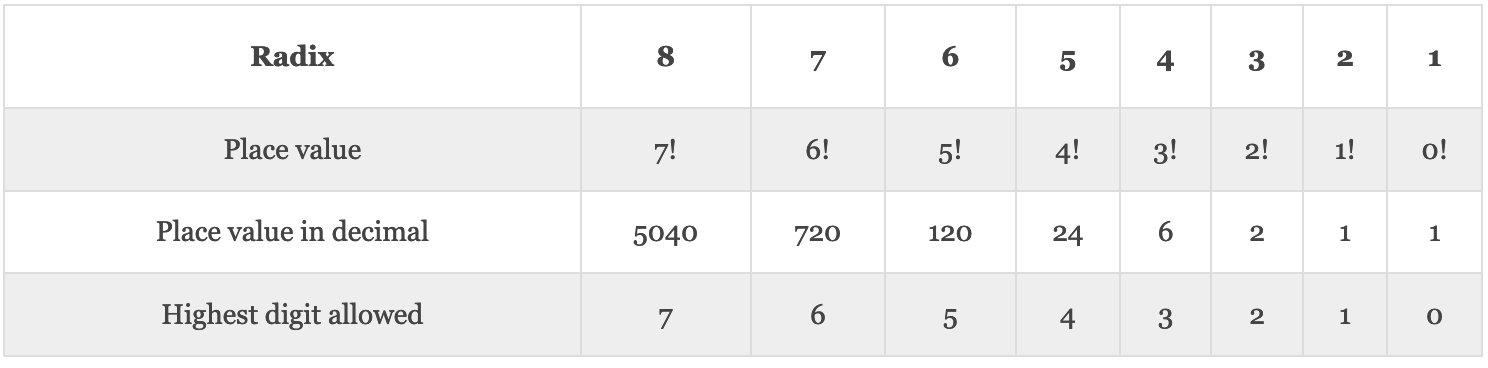To convert a value from decimal to factoradic, we just need to take the integer division of the value by the radix-starting from 1, 2, 3,… During the successive divisions, we keep track of the remainders–until the division results in 0. The remainder of each division will correspond to the factoradic number digit at that position, going from right to left. For example, to convert 256 (decimal) to factorial number representation:
要将值从十进制转换为乘数，我们只需要对值进行整数除以从1、2、3等开始的基数即可。在连续除法过程中，我们会跟踪余数–直到除法结果为0 。 每个除法的其余部分将对应于该位置从右到左的分位数数字。 例如，要将256 (十进制)转换为阶乘数表示形式：
256 / 1 = 256, remainder 0 256 / 2 = 128, remainder 0 128 / 3 =  42, remainder 2  42 / 4 =  10, remainder 2  10 / 5 =   2, remainder 0   2 / 6 =   0, remainder 2
Arranging the remainders–starting from the bottom and going up–we get the factoradic number: 202200. As an exercise, you can try to convert it back to decimal representation using the table above. We just need to multiply the digit at each position by its corresponding place value and then add them all up.
从底部开始向上排列其余部分，我们得到因数 ： 202200 。 作为练习，您可以尝试使用上表将其转换回十进制表示形式。 我们只需要将每个位置的数字乘以其对应的位置值 ，然后将它们加起来即可。
使用阶乘数系统查找第N个置换 (Using the Factorial Number System to Find the Nth Permutation)
We are now ready to use the factorial number system to help us find our arbitrary permutation in the sequence. It turns out there’s a relationship between this number system and the lexicographically ordered permutations of a set. This relationship or mapping is known as the Lehmer code.
现在，我们准备使用阶乘数系统来帮助我们找到序列中的任意排列。 事实证明，此数字系统与集合的按字典顺序排列的排列之间存在关系。 这种关系或映射称为Lehmer码 。
Now, let’s go back to our question of finding the 980,000th permutation in the lexicographically ordered permutations of the set {0 1 2 3 4 5 6 7 8 9}. As you can see, the set is initially sorted (in non-decreasing order). This represents the lowest permutation of the set. Remember that with this method, we don’t need to generate all previous permutations to get to the one we’re looking for.
现在，让我们回到在集合{0 1 2 3 4 5 6 7 8 9}的按字典顺序排列的排列中找到第980,000个排列的问题。 如您所见，该集合首先进行了排序(以非降序排列)。 这表示该集合的最低置换。 请记住，使用这种方法，我们不需要生成所有先前的排列就可以找到我们想要的排列。
We proceed as follows. First, we are going to assign an index–starting from 0–to all the digits in the set. Initially, index 0 corresponds to digit 0, index 1 to digit 1, and so on, up to index 9 corresponding to digit 9. Also, we’ll enumerate each permutation in the sequence, starting from 0. So, the initial ordering of the set is permutation number 0. Therefore, we now need to find the (980,000th-1) = 979,999th permutation.
我们进行如下。 首先，我们将为索引集中的所有数字分配一个从0开始的索引。 最初，索引0对应于数字0，索引1至位1，以此类推，直到对应于数字9索引9。 同样，我们将从0开始枚举序列中的每个排列。 因此，集合的初始排序为排列号0 。 因此，我们现在需要找到第(980,000th-1)= 979,999个排列。
Now, we take the number 979,999 and start by diving it by (n -1)! where n is the number of elements in the set-in our case, n = 10–so we begin dividing by 9!. We will keep track of the remainder and the integer quotient:
现在，我们将数字979,999乘以(n -1)开始！ 在这里， n是集合中元素的数量， n = 10–因此我们开始除以9！ 。 我们将跟踪余数和整数商：
Initial set: {0 1 2 3 4 5 6 7 8 9} 979,999 / 9! = 2, remainder 254,239 permutation 1st digit: 2
Here, the result of the integer division, 2, indicates the index–inside our set–of the number that corresponds to the first digit in our 979,999th permutation. Index 2 corresponds to number 2. We now take this number out of the set, take the remainder as our new numerator, and continue to the next iteration, subtracting 1 from n in the denominator (n-1)!. We will continue this process until, and including, (n -1)! = 0!:
在这里，整数除法2的结果表示与我们的第979,999个排列中的第一个数字相对应的数字的索引(在我们的集合之内)。 索引2对应于数字2 。 现在，我们从集合中取出该数字，将余数作为新分子，然后继续进行下一个迭代，从分母(n-1)的 n中减去1 ！ 。 我们将继续此过程，直到并包括(n -1)！ = 0！ ：
set: {0 1 3 4 5 6 7 8 9} 254,239 / 8! = 6, remainder 12,319 permutation 2nd digit: 7 set: {0 1 3 4 5 6 8 9} 12,319 / 7! = 2, remainder 2,239 permutation 3rd digit: 3 set: {0 1 4 5 6 8 9} 2,239 / 6! = 3, remainder 79 permutation 4th digit: 5 set: {0 1 4 6 8 9} 79 / 5! = 0, remainder 79 permutation 5th digit: 0 set: {1 4 6 8 9} 79 / 4! = 3, remainder 7 permutation 6th digit: 8 set: {1 4 6 9} 7 / 3! = 1, remainder 1 permutation 7th digit: 4 set: {1 6 9} 1 / 2! = 0, remainder 1 permutation 8th digit: 1 set: {6 9} 1 / 1! = 1, remainder 0 permutation 9th digit: 9 set: {6} 0 / 0! = 0, remainder 0 permutation 10th digit: 6
Putting all digits together, we get: {2 7 3 5 0 8 4 1 9 6}. This is the 980,000th permutation in lexicographic order of our set.
将所有数字放在一起，我们得到： {2 7 3 5 0 8 8 4 1 9 6} 。 这是我们集合中按字典顺序排列的第980,000个排列。
Notice that the result of each integer division above corresponds to each digit in the factoradic number representation of 979,999 decimal. Putting these digits together gives 2623031010. This is the factorial number representation of 979,999 decimal:
请注意，上面每个整数除法的结果对应于979999小数的乘数表示中的每个数字。 将这些数字放在一起得出2623031010 。 这是979999小数的阶乘数表示形式 ：
979,000(decimal) = 2623031010(factoradic)
This illustrates the mapping between the factorial number system and lexicographic permutations. And, equivalently, we could’ve started by converting 979,000–following the procedure in the previous section–to its factorial number representation. Then use each digit in the factoradic number as an index to our shrinking set, as we did above.
这说明了阶乘数系统和词典编排之间的映射。 同样，我们可以按照上一节中的步骤将979,000转换为其阶乘数表示形式。 然后，像上面一样，将乘数中的每个数字用作收缩集合的索引。
排列的应用 (Applications of Permutations)
In this article, we went from the basic definitions of a permutation to discussing different ways of generating them. We were particularly interested in generating permutations in lexicographic order, and how we can do it efficiently. To finalize, I’ll briefly mention some applications of permutations in different areas.
在本文中，我们从排列的基本定义转到讨论生成排列的不同方法。 我们对按字典顺序生成排列以及如何高效地进行排列特别感兴趣。 最后，我将简要介绍一下排列在不同区域中的一些应用。
Some of these applications include error detection and correction algorithms in information theory. Permutations are used to analyze sorting algorithms in computer science. They are also used to describe RNA sequences in biology. And, they appear in many branches of mathematics.
其中一些应用包括信息论中的错误检测和纠正算法。 排列用于分析计算机科学中的排序算法。 它们还用于描述生物学中的RNA序列。 而且，它们出现在数学的许多分支中。
Hopefully, this discussion of permutations gave you another perspective and inspired you to continue exploring the subject.
希望这种对排列的讨论为您提供了另一种观点，并启发您继续探索该主题。

Originally published at https://stemhash.com on August 31, 2020.
最初于 2020年8月31日 发布于 https://stemhash.com 。

翻译自: https://levelup.gitconnected.com/efficient-permutations-in-lexicographic-order-8e004f94b4b8

将字典按某顺序排列

展开全文python leetcode
• Python三个数字按照从大到小的顺序排列，在线等回答 Python三个数字按照从大到小的顺序排列，在线等回答开发语言
• 1.一开始样式用的plain，是按照字母的顺序排列的，比较次序为作者，年度和标题；想换成引用顺序； 百度大法后，用了unsrt;但是标号还是没变化。 2.解决办法： 最后，附上Elsarticle的模板链接：...latex
• //将三个数字按照从小到大的顺序排列 void Sort(int &a, int &b, int &c); //交换数据 void Change(int &a, int &b); void main() { int a, b, c; cout请输入三个数字:"; cin>>a; cin>>b; cin>>c;
• 即是按照有小到大的顺序排列。 2.元素为引用类型 当元素为引用类型，排列顺序按照元素内的某个变量值大小来排序。规则和上面一样。代码如下： orders.Sort((x, y) => x.angle.CompareTo(y.angle));//有小...
• 如果要将数组中的数字按照从小到大的顺序排列。 完成思路：将最小的数字放在数组的第一项，将第二小的数组放在第二项，一直重复，知道完成。 那么如何将最小的数字放在数组索引为0的位置呢？ 示例： for（int ...
• //本题的思路：要在“123456789”中添加加减号，那么可以将它们隔开可以是这样“ 1 2 3 4 5 6 7 8 9”，这样在他们的间隙就有空格（包括最前面的空格）9个，而这些空//格可以填写的内容只有3种情况：1....
• Talk is cheap,show me code~穷举法
• 4.7 编写一个递归函数，将所输入的 10 个字符相反顺序排列出来。
• //输入5个数(含负数、小数)将它们由小到大的顺序排列起来 //提示：需要排数的数字通过参数传递进来， #include float paixu(float b);//声明paixu函数 int main() { float a;//存放5个数包括...
• a=input('请输入第一个数值：'); b=input('请输入第二个数值：'); c=input('请输入第三个数值：'); m=0; if a<b m=a; a=b; b=m; end if a<c m=a; a=c; c=m; end if b<c m=c;......
• 题目:输入 5 个数(含负数、小数)将它们由小到大的顺序排列起来。 提示：需要排序的数字通过参数传递进来。 例如： 输入：-1 2.1 -3 5 7 输出: -3 -1 2.1 5 7import java.util.Scanner;public class ...
• 要求移动数码，最后使得数码12345678的顺序顺序排列
• import java.util.ArrayList; import java.util.Collections; public class Test1 { public static void main(String[] args) { ArrayList c = new ArrayList(); c.add(1); c.add(2);... c.add(4java
• 主要功能：用选择排序从小到大的顺序排列数 #include &amp;amp;lt;stdio.h&amp;amp;gt; #define N 10 void SelectionSort(int *a,int n) { int i,j,temp; for(i=0;i&amp;amp;lt;n-1;i++) { for(j=...C语言 算法设计 选择排序源码
• 前端页面的列表序号的情况是这样的， 杂乱无章 在前端代码的 有一个colums,是显示表格内容的 在表格内容栏加上 {prop:"", label:"序号",type:"index"}, 就可以实现列表的序号顺序排列vue vue.js
• 之前使用fastjson的1.1.32版本，将字符串转json，然后toString后，各key是字母顺序排列的，如下所示： JSONObject obj = JSONObject.parseObject("{\"command\":\"www\",\"sesID\":\"123456789\",\"content\":\...json
• 以输入十个数为例： //终曲cc-学习之路 #include <stdio.h> int main() { int a; printf("请输入10个数：\n"); for(int i=0;i<=9;i++) { scanf("%d",&a[i]); ... intc语言 排序算法
• newArr.append(arr.pop(smallest))#每一次都findSmallest里面的最小值删除并存放在新的数组newArr中 return newArr print(selectionSort([5, 3, 6, 2, 10])) 运行结果如下： [2, 3, 5, 6, 10]
• 英文字符及数字字母排列顺序为： ! # \$ % & ( ) , . ' - ; @ [ ] ^ _ ` { } ~ + = 0 1 2 … 9 A B C … Z 系统不区分大小写字母 刚才测试了一下中文字符，日语假名，汉字，部分其他语种等 中文英文日文字符
• 输入数据有多组，每组占一行，每行的第一个数字为n,接着是n个整数，n=0表示输入数据的结束，不做处理。 Output 对于每个测试实例，输出排序后的结果，两个数之间用一个空格隔开。每个测试实例占一行。 Sample Inpu
• 不能直接比较内存中的数字，所以我们需要将其中一个数字放到寄存器AX中 CMP AX,DATA1[SI+2] ;比较两个数 JLE L3 ;小于等于的话，则直接跳到下一对数据的比较 XCHG AX,DATA1[SI+2] ;若大于，则通过两个XCHG语句，交换...排序算法 算法 编程语言...